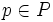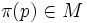# Connection on vector bundle equals connection on principal GL-bundle

## Statement

### Conceptual statement

We know that given a differential manifold, the vector bundles of dimension$r$ over that manifold are in one-one correspondence with the principal$GL(r)$-bundles over the manifold.

It turns out under this correspondence, the notion of connection on the vector bundle, corresponds to the notion of connection on the corresponding principal$GL(r)$-bundle.

### Ordinary statement

Let$M$ be a differential manifold and$E$ be a$r$-dimensional vector bundle over$M$. Suppose$P$ is the corresponding principal$GL(r)$-bundle over$M$. Then, there is a natural bijection between the set of connections on$E$ (viewed as a vector bundle) and the set of connections on$P$ (viewed as a principal$GL(r)$-bundle).

## Definitions used

### Connection on a vector bundle

Further information: Connection on a vector bundle

### Connection on a principal bundle

Further information: Connection on a principal bundle

## Proof

### From connection on a vector bundle, to connection on principal bundle

Suppose$E$ is a$r$-dimensionalvector bundle on the differential manifold$M$, and$\nabla$ is a connection on$E$. Let$\pi:P \to M$ be the corresponding principal$GL(r)$-bundle.

Here's how we use$\nabla$ to get a connection on the principal$GL(r)$-bundle. Observe, first, that giving a point$p \in P$ is equivalent to specifying a point$\pi(p) \in M$, and a basis for the tangent space at$\pi(p)$. Further, giving a tangent vector$v$ at the point$p \in P$, then Fill this in later